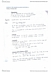# MATH137 Lecture Notes - Lecture 1: Inverse Trigonometric Functions, Algebraic Function, Transcendental Function

30 views20 pages
School
Department
Courseyellowelk480 and 36439 others unlocked36
Verified Note
36 documents

## Document Summary

Math 137 week 1 notes dr. paula smith: appendix a, numbers: counting, whole, integers, rational, irrational, transcendental, real. Z = {integers} = {0, 1, 2, } N = {positive integers} = {1, 2, 3, : intervals. Open: (a, b) = {x | a < x < b} Closed: [a, b] = {x | a x b} Half-open: [a, b) = {x | a x < b} b or (a, b] = {x | a < x b} Do not confuse the open interval (a,b) with the point (a, b) 2: inequalities. [a, ) = {x | x a} ( , b) = {x | x < b} This is shorthand for goes on without bound. b b a a a b a a a a b b b b. {x | x > b} (b, ) or {x | x < a} ( , a)

## Get access

\$8 USD/m\$10 USD/m
Billed \$96 USD annuallyHomework Help
Study Guides
Textbook Solutions
Class Notes
Textbook Notes
Booster Class
Class+
\$8 USD/m
Billed \$96 USD annuallyHomework Help
Study Guides
Textbook Solutions
Class Notes
Textbook Notes
Booster Class